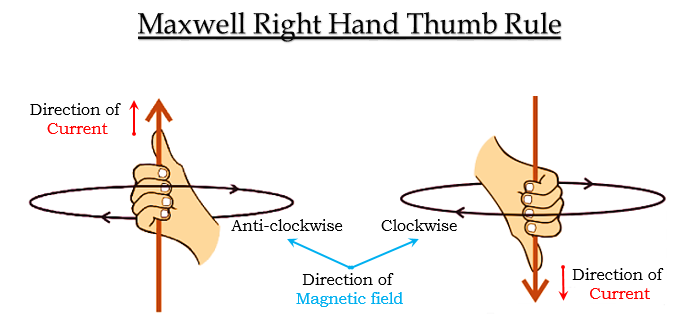# In the straight wire A, current is flowing in the vertically downwards direction whereas in wire B the current is flowing in the vertically upward direction. What is the direction of magnetic field:(a) in wire A?(b) in wire B?Name the rule which you have used to get the answer.

We will use the right-hand thumb rule to get the answer.

(a) In the straight wire A, the current is flowing in vertically downwards direction, therefore, the direction of the magnetic field around it will be clockwise.

(b) In the straight wire B, the current is flowing in vertically upwards direction; therefore, the direction of the magnetic field around it will be anticlockwise.

Extra information with the image is for reference only

According to Maxwell’s right-hand thumb rule, when a current-carrying conductor is held in the right hand so that our thumb points in the direction of the current, and the fingers that have wrapped the conductor gives the direction of the magnetic field lines around the wire.

When thumb points upwards (current flowing vertically upwards), the wrapped fingers are anticlockwise. Hence, the direction of the magnetic field is anticlockwise.

While when thumb points downwards (current flowing vertically downwards), the wrapped fingers are clockwise. Hence, the direction of the magnetic field is clockwise.Updated on: 10-Oct-2022

406 Views